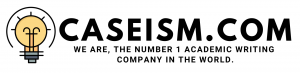# Milk and Money Case Solution & Answer

Question 2

The mailbox price is the price at which the farmers used to sell simple milk in the market.To understand which commodityâ€™s price is more closely related to the mailbox prices,we mustunderstand the relationship between those two variables.It is also important to calculate the correlation between them.

Correlation is the method by which calculates the relationship between the two independent variables. The test result of the correlation is between +1 and -1. That means the result must be near to the +1; thereis astrong positive relationship between the two variables.

If one variable increases, then other will also increase in response and ifit decreases, then other would also decrease.This shows apositive relationship between the variables. Similarly, if the test result is near to -1, or is negative, then it shows that if one variable increases then, other would decrease. If one variable decreases, then other would increase in another direction. There is anegative correlation between the two variables.

Similarly, see Exhibit 4. Which shows that Class IV has acorrelation of 0.84, and Class III has correlation score of 0.96, Butter has 0.79,and finally the NFDM is 0.17. However, if we take a look at what the price of acommodity that is strongly and positively correlated with the price of the mailbox, then the category would have a high score. In this case itwould be Class III with ascore of0.96, which is thehighestscore and shows astrong positive relationship with the mailbox price.

Question 3 (premium \$0.40, and fees and commission is \$0.05)

Gerard should buy a put option. Which is a contract that gives right to sell the commodity at the agreed price in future. However, the put option is flexible, because it is not anobligation on the purchaser of the option to sell, but he can let the contract expire in such a situation when exercising the option would bring aloss for the exerciser.

Meanwhile, the most closely related commodity to Gerard is Class III, so he should purchase a put option at the strike price of the \$13.6. Which means that if the commodity prices decrease,Â  Gerard can optÂ  to sell. He would be able to sell that commodity at the strike price of \$13.6, no matter what is the current price of that commodity in the market. See Exhibit 5

Question 4

The strike price was \$13.6 in this situation. Whereas,assuming that market price of mailbox would be \$12.5. In this sense, the payoff of the option is \$1.1. Indeed it is profitable before fees and commission. Therefore, if the mailbox price in the current month is assumed at \$11.5, then we calculate thenet price. It would be (mailbox price + value) = 11.5+1.1=12.6. Which exceeds the \$12.5.

Question 5

The premium cost of the option is \$0.4, fees and commission of the option are \$0.05. Meanwhile, the total cost of theoption is \$0.45. If we add the cost of the option to the value calculated in the last question which is \$12.6, we get (12.6+0.45=13.05). That means the net price would be \$13.05…………….

This is just a sample partical work. Please place the order on the website to get your own originally done case solution.